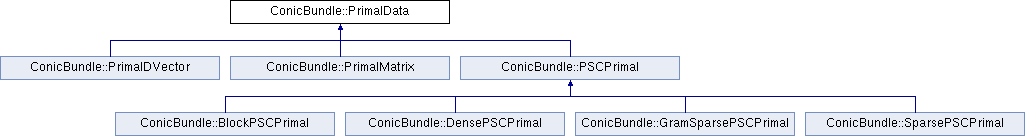# ConicBundle::PrimalData Class Reference [Interface to ConicBundle for the Language C++]

In Lagrangean relaxation an approximate primal solution can be generated by supplying primal information derived from this abstract class for each epsilon subgradient within ConicBundle::FunctionOracle::evaluate(). More...

`#include <CBSolver.hxx>`

Inheritance diagram for ConicBundle::PrimalData:List of all members.

## Public Member Functions

virtual PrimalDataclone_primal_data ()=0
Returns a newly generated identical Object.
virtual int assign_primal_data (const PrimalData &pd)=0
Copy the information of pd to *this.
virtual int aggregate_primal_data (double myfactor, double itsfactor, const PrimalData &it)=0
multiply *this with myfactor and add itsfactor*it to this

## Detailed Description

In Lagrangean relaxation an approximate primal solution can be generated by supplying primal information derived from this abstract class for each epsilon subgradient within ConicBundle::FunctionOracle::evaluate().

In many applications, e.g. in Lagrangean relaxation, the convex minimization problem arises as the dual of a convex primal maximization problem. In this case one is typically interested in obtaining a primal approximate solution in addition to the dual solution. Under reasonable conditions this is possible if the primal solutions that give rise to the subgradients are aggregated along with the subgradients maintained by the bundle algorithm. Within the interface the user can achieve this by specifying and returning in the oracle an appropriate derivation of the class ConicBundle::PrimalData. The classical case of a primal ConicBundle::DVector is supplied as an example implementation below.

The primal data has to be supplied within ConicBundle::FunctionOracle::evaluate() and can be retrieved via the methods ConicBundle::CBSolver::get_approximate_primal() and ConicBundle::CBSolver::get_center_primal()

The documentation for this class was generated from the following file:

Generated on Mon Nov 8 19:36:41 2010 for ConicBundle by1.5.6